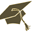Thesis (Selection of subject)(version: 290)
Assignment details
Login via CASO původu nevratných procesů
Thesis title in Czech: O původu nevratných procesů On the origin of irreversible processes Nevratnost, entropie, disipace, hamiltonovská evoluce, samoregularizace Irreversibility, entropy, dissipation, Hamiltonian evolution, self-regularization 2018/2019 diploma thesis Mathematical Institute of Charles University (32-MUUK) RNDr. Michal Pavelka, Ph.D.
 Guidelines 1) Formulation of classical mechanics, rigid body motion and reversible fluid mechanics in the Hamiltonian setting (using a Poisson bracket). 2) Self-regularization of classical mechanics using the approach from . Numerical examples. 3) Self-regularization of rigid body motion using the approach from . Numerical examples. 4) Self-regularization of fluid mechanics using the approach from . Numerical examples. 5) Discussion of Landau damping . 6) Discussion of the origin of the second law of thermodynamics comparing  and .
 References  Michal Pavelka, Václav Klika and Miroslav Grmela, Statistical mechanics of Landau damping, Submitted to Entropy (2018)
 Preliminary scope of work in English Hamilton canonical equations, which describe evolution within classical mechanics, are completely reversible. Assuming that macroscopic systems around us can be approximated as systems of classical particles, evolution of the systems can be also described by reversible classical mechanics. On the other hand, processes that the macroscopic systems undergo are typically irreversible (friction, diffusion, temperature equilibration, etc.). The question is from where this irreversibility comes from. Why does entropy of an isolated system grow in time? What is the reason behind the second law of thermodynamics?

Charles University | Information system of Charles University | http://www.cuni.cz/UKEN-329.html